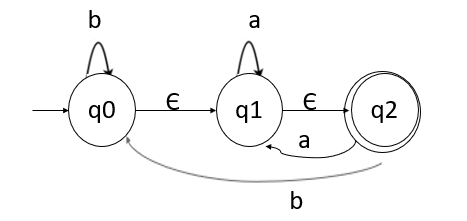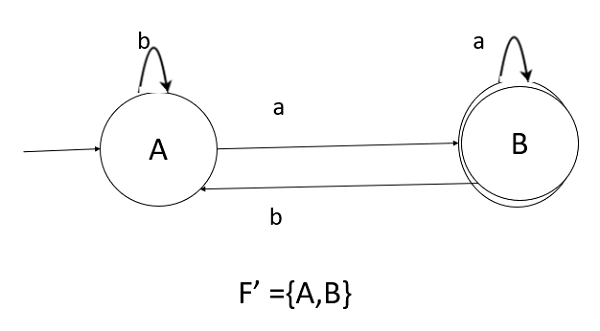# How to convert NFA with epsilon to DFA in TOC?

In this method, we first convert Non-deterministic finite automata (NFA) with ε to NFA without ε.

Then, NFA without ε- can be converted to its equivalent Deterministic Finite Automata (DFA).

## Method for conversion

The method for converting the NFA with ε to DFA is explained below −

Step 1 − Consider M={Q, Σ, δ,q0,F) is NFA with ε. We have to convert this NFA with ε to equivalent DFA denoted by

M0=(Q0,Σ, δ0,q0,F0)

Then obtain,

ε-closure(q0) ={p1,p2,p3,……pn}

then [p1,p2,p2,….pn] becomes a start state of DFA

now[p1,p2,p3,….pn] ∈ Q0

Step 2 − We will obtain δ transition on [p1,p2,p3,…pn] for each input.

δ 0([p1,p2,p3,..pn],a) = ε-closure(δ(p1,a) U δ(p2,a2)U……………… δ(pn,a))

= U (i=1 to n) ε-closure d(pi,a)

Where a is input ∈Σ

Step 3 − The state obtained [p1,p2,p3,…pn] ∈ Q0 .

The states containing final state in pi is a final state in DFA

## Example

Convert the following NFA with epsilon to equivalent DFA

## Solution

Consider the following NFA for conversion of NFA with epsilon to DFA −To convert this NFA with epsilon, we will first find the ε-closures, as given below −

• ε-closure(q0)={q0,q1,q2}
• ε-closure(q1)={q1,q2}
• ε-closure(q2)={q2}

Let us start from ε-closure of start state, as mentioned below −

When, ε-closure(q0)={q0,q1,q2}, we will call this state as A.

Now, let us find transition on A with every input symbol, as shown below −

δ'(A, a) = ε-closure(δ(A,a))
= ε-closure(δ(q0,q1,q2), a))
= ε-closure(δ(q0, a) ∪ δ(q1,a) U δ(q2,a) )
= ε-closure(ΦUq1 ∪q2)
= ε-closure(q1)
= {q1, q2} let us call it as state B
δ'(A, b) = ε-closure(δ(A,b))
= ε-closure(δ(q0,q1,q2), b))
= ε-closure(δ(q0, b) ∪ δ(q1,b) U δ(q2,b) )
= ε-closure(q0 U Φ∪q0)
= ε-closure(q0)
= {q0,q1, q2} its nothing but state A
δ'(B, a) = ε-closure(δ(B,a))
= ε-closure(δ(q1,q2), a))
= ε-closure(δ(q1,a) U δ(q2,a) )
= ε-closure(q1 ∪q2)
= ε-closure(q1)
= {q1, q2} its nothing but state B
δ'(B, b) = ε-closure(δ(B,b))
= ε-closure(δ(q1,q2), b))
= ε-closure(δ(q1,b) U δ(q2,b) )
= ε-closure(Φ∪q0)
= ε-closure(q0)
= {q0,q1, q2} its nothing but state A

Hence, the transition table for the generated DFA is as follows −

States\inputsab
ABA
BBA

The DFA diagram is as follows• As, A={q0,q1,q2} in which the final state q2 lies. Hence, A is the final state.
• In B ={q1,q2} the state q2 lies. Hence, B is also the final state.